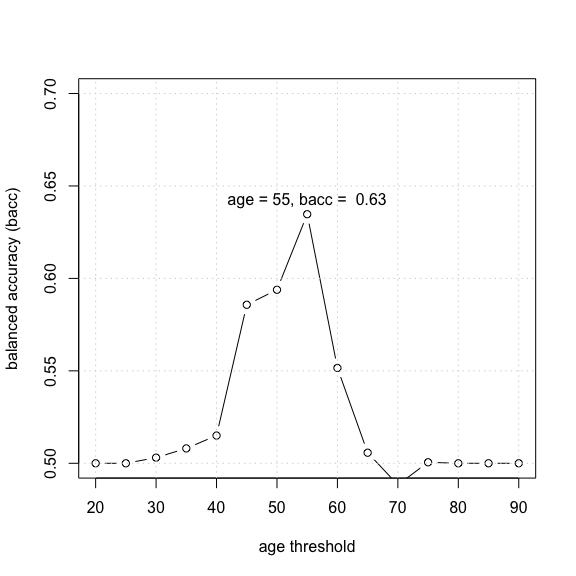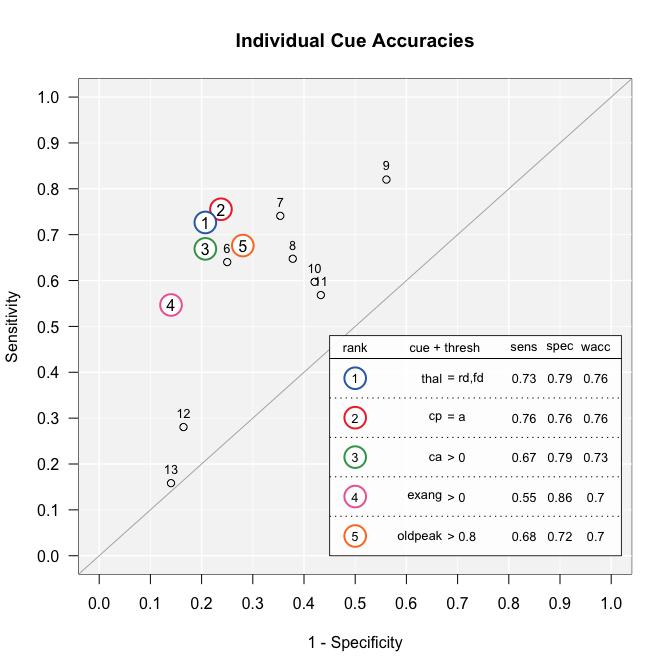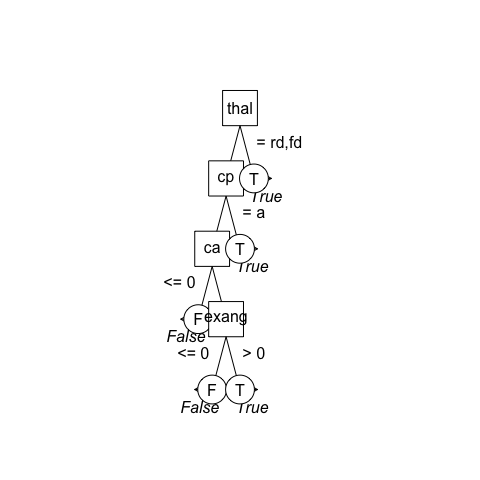# FFTrees tree construction algorithms

#### 2018-09-15

FFTrees currently contains 4 different tree construction algorithms:

Algorithm Full Name Reference
ifan Marginal Fan Phillips, Neth, Gaissmaier, & Woike (2017)
dfan Conditional Fan Phillips et al. (2017)
zigzag Zig-Zag Martignon, Katsikopoulos, & Woike (2008)
max Max Martignon et al. (2008)

## ifan

The default algorithm used to create trees "ifan" can be summarised in five steps.

5 Steps in growing FFTs using the ifan algorithm.
Step Function Description
1 cuerank For each cue, calculate a classification threshold that maximizes goal.chase (default is bacc) of classifications of all data based on that cue (that is, ignoring all other cues). If the cue is numeric, the threshold is a number. If the cue is a factor, the threshold is one or more factor levels.
2 grow.FFTrees() Rank cues in order of their highest balanced accuracy value calculated using the classification threshold determined in step 1
3 grow.FFTrees() Create all possible trees by varying the exit direction (left or right) at each level to a maximum of X levels (default of max.levels = 4).
4 grow.FFTrees() Reduce the size of trees by removing (pruning) lower levels containing less than X% (default of stopping.par = .10) of the cases in the original data.
5 grow.FFTrees() Select the FFT with the highest goal (default is bacc value as the final tree (tree.max)

## Example: Heart Disease

First, we’ll calculate a classification threshold for each cue using cuerank():

heartdisease.ca <- cuerank(formula = diagnosis ~.,
data = heartdisease)

# Print key results
heartdisease.ca[c("cue", "threshold", "direction", "bacc")]
##         cue            threshold direction  bacc
## 1       age                   55         > 0.635
## 2       sex                    0         > 0.630
## 3        cp                    a         = 0.759
## 4  trestbps                  140         > 0.558
## 5      chol                  242         > 0.568
## 6       fbs                    0         > 0.509
## 7   restecg hypertrophy,abnormal         = 0.588
## 8   thalach                  148        <= 0.695
## 9     exang                    0         > 0.703
## 10  oldpeak                  0.8         > 0.698
## 11    slope            flat,down         = 0.694
## 12       ca                    0         > 0.731
## 13     thal                rd,fd         = 0.760

Here, we see the best decision threshold for each cue that maximizes its balanced accuracy (bacc) when applied to the entire dataset (independently of other cues). For example, for the age cue, the best threshold is age > 55 which leads to a balanced accuracy of 0.63. In other words, if we only had the age cue, then the best decision is: “If age > 55, predict heart disease, otherwise, predict no heart disease”.

Let’s confirm that this threshold makes sense. To do this, we can plot the bacc value for all possible thresholds as in Figure @ref(fig:agethreshold):Plotting the balanced accuracy of each decision threshold for the age cue.

Next, the cues are ranked by their balanced accuracy. Let’s do that with the heart disease cues:

# Rank heartdisease cues by balanced accuracy
heartdisease.ca <- heartdisease.ca[order(heartdisease.ca$bacc, decreasing = TRUE),] # Print the key columns heartdisease.ca[c("cue", "threshold", "direction", "bacc")] ## cue threshold direction bacc ## 13 thal rd,fd = 0.760 ## 3 cp a = 0.759 ## 12 ca 0 > 0.731 ## 9 exang 0 > 0.703 ## 10 oldpeak 0.8 > 0.698 ## 8 thalach 148 <= 0.695 ## 11 slope flat,down = 0.694 ## 1 age 55 > 0.635 ## 2 sex 0 > 0.630 ## 7 restecg hypertrophy,abnormal = 0.588 ## 5 chol 242 > 0.568 ## 4 trestbps 140 > 0.558 ## 6 fbs 0 > 0.509 Now, we can see that the top five cues are thal, cp, ca, thalach and exang. Because ffts rarely exceed 5 cues, we can expect that the trees will use a subset (not necessarily all) of these 5 cues. We can also plot the cue accuracies in ROC space using the showcues() function: # Show the accuracy of cues in ROC space showcues(cue.accuracies = heartdisease.ca)Cue accuracies for the heartdisease dataset. The top 5 cues in terms of balanced accuracy are highlighted. Next, grow.FFTrees() will grow several trees from these cues using different exit structures: # Grow FFTs heartdisease.ffts <- grow.FFTrees(formula = diagnosis ~., data = heartdisease) # Print the tree definitions heartdisease.ffts$tree.definitions
##   tree nodes classes             cues directions  thresholds     exits
## 1    1     3   c;c;n       thal;cp;ca      =;=;>   rd,fd;a;0   1;0;0.5
## 2    2     4 c;c;n;n thal;cp;ca;exang    =;=;>;> rd,fd;a;0;0 1;0;1;0.5
## 3    3     3   c;c;n       thal;cp;ca      =;=;>   rd,fd;a;0   0;1;0.5
## 4    4     4 c;c;n;n thal;cp;ca;exang    =;=;>;> rd,fd;a;0;0 1;1;0;0.5
## 5    5     4 c;c;n;n thal;cp;ca;exang    =;=;>;> rd,fd;a;0;0 0;0;1;0.5
## 6    6     4 c;c;n;n thal;cp;ca;exang    =;=;>;> rd,fd;a;0;0 1;1;1;0.5
## 7    7     4 c;c;n;n thal;cp;ca;exang    =;=;>;> rd,fd;a;0;0 0;0;0;0.5

Here, we see that we have 7 different trees, each using some combination of the top 5 cues we identified earlier. For example, tree 1 uses the top 4 cues, while tree 3 uses only the top 3 cues. Why is that? The reason is that the algorithm also prunes lower branches of the tree if there are too few cases classified at lower levels. By default, the algorithm will remove any lower leves that classfify fewer than 10% of the original cases. The pruning criteria can be controlled using the stopping.rule, stopping.par and max.levels arguments in grow.FFTrees()

Now let’s use the wrapper function FFTrees() to create the trees all at once. We will then plot tree #4 which, according to our results above, should contain the cues thal, cp, ca"

# Create trees
heart.fft <- FFTrees(formula = diagnosis ~.,
data = heartdisease)

# Plot tree # 4
plot(heart.fft,
stats = FALSE,    # Don't include statistics
tree = 4)## "dfan"

The "dfan" algorithm is identical to the "ifan" algorithm with one (important) exception: In algorithm = "dfan", the thresholds and rankings of cues are recalculated for each level in the FFT conditioned on the exemplars that were not classified at higher leves in the tree. For example, in the heartdisease data, using algorithm = "dfan" would first classify some cases using the thal cue at the first level, and would then calculate new accuracies for the remaining cues on the remaining cases that were not yet classified. This algorithm is appropriate for datasets where cue validities systematically differ for different (and predictable) subsets of data. However, because it calculates cue thresholds for increasingly smaller samples of data as the tree grows, it is also, potentially, more prone to overfitting compared to algorithm = "ifan"

## Additional arguments

One can adjust the "ifan" and "dfan" algorithms in multiple ways. The most important arguments are goal and goal.chase which affect how thresholds are determined for each cue, and how cues are selected and ordered.

Argument Functionality Default Other possible arguments
goal.chase Specifies which statistic is optimized when calculating cue thresholds and ranking cues. goal.chase = "bacc" "wacc", "acc", "dprime", "cost"
goal Specifies which statistic is optimized when selecting an FFT in the fan. goal = "wacc" "acc", "dprime", "cost"

## Specifying costs

If goal = "cost" and/or goal.chase = "cost", the fan algorithms will try to minimize costs. One can specify two types of costs, cost.cues, the cost of using a cue to classify a case,, and cost.outcomes the cost of different outcomes.

cost.cues should be a data frame with two columns, one column giving the names of cues with costs, and one column giving the actual costs. For example, in the heartdisease dataset, cues have the following costs:

heart.cue.cost <- list(age = 1,
sex = 1,
cp = 1,
trestbps = 1,
chol = 7.27,
fbs = 5.2,
restecg = 15.5,
thalach = 102.9,
exang = 87.3,
oldpeak = 87.3,
slope = 87.3,
ca = 100.9,
thal = 102.9)

cost.outcome should be a vector of length 4 indicating the cost of hits, false alarms, misses, and correct rejections reespectively. For example, if a false alarm has a cost of $500 and a miss has a cost of$1000, one could specify:

# Specify the following costs for heart disease diagnosis:
# cost(Hit) = 0, cost(False Alarm) = 100, cost(Miss) = 200, cost(correct rejection) = 0

heart.cost.outcomes <- list(hi = 0, fa = 500, mi = 1000, cr = 0)

Here is an FFT that is built with the goal of maximizing balanced accuracy and ignoring these costs:

heart.costA.fft <- FFTrees(formula = diagnosis ~.,
data = heartdisease,
cost.outcomes = heart.cost.outcomes,
cost.cues = heart.cue.cost,
goal = "bacc",
goal.chase = "bacc")

Let’s look at the performance of the best performing tree. The best tree has bacc = 0.812 and cost = 252

summary(heart.costA.fft)
##        train test
## n    303.000   NA
## hi   118.000   NA
## mi    21.000   NA
## fa    37.000   NA
## cr   127.000   NA
## mcu    1.733   NA
## pci    0.876   NA
## cost 251.800   NA
## acc    0.809   NA
## bacc   0.812   NA
## sens   0.849   NA
## spec   0.774   NA

Now, we can build an FFT that tries to respect these costs:

heart.costB.fft <- FFTrees(formula = diagnosis ~.,
data = heartdisease,
cost.outcomes = heart.cost.outcomes,
cost.cues = heart.cue.cost,
goal = "cost",
goal.chase = "cost")

Here’s it’s performance: it has a slightly lower balanced accuracy of bacc = 0.76, but a much lower cost of cost = 160

summary(heart.costB.fft)
##        train test
## n    303.000   NA
## hi   133.000   NA
## mi     6.000   NA
## fa    72.000   NA
## cr    92.000   NA
## mcu    2.056   NA
## pci    0.853   NA
## cost 160.512   NA
## acc    0.743   NA
## bacc   0.759   NA
## sens   0.957   NA
## spec   0.561   NA

## "max""zigzag"

The max and zigzag algorithms are described in Martignon et al. (2008).